# Heptacontagon

Regular heptacontagon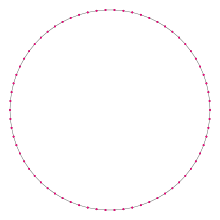A regular heptacontagon
Type Regular polygon
Edges and vertices 70
Schläfli symbol {70}, t{35}
Coxeter diagramSymmetry group Dihedral (D70), order 2×70
Internal angle (degrees) ≈174.9°
Dual polygon Self
Properties Convex, cyclic, equilateral, isogonal, isotoxal

In geometry, a heptacontagon (or hebdomecontagon from Ancient Greek ἑβδομήκοντα, seventy) or 70-gon is a seventy-sided polygon. The sum of any heptacontagon's interior angles is 12240 degrees.

A regular heptacontagon is represented by Schläfli symbol {70} and can also be constructed as a truncated triacontapentagon, t{35}, which alternates two types of edges.

## Regular heptacontagon properties

One interior angle in a regular heptacontagon is 17467°, meaning that one exterior angle would be 517°.

The area of a regular heptacontagon is (with t = edge length)The circumradius of a regular heptacontagon isSince 70 = 2 × 5 × 7, a regular heptacontagon is not constructible using a compass and straightedge, but is constructible if the use of an angle trisector is allowed.

## Symmetry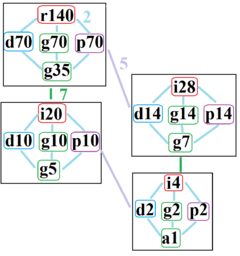The symmetries of a regular heptacontagon. Light blue lines show subgroups of index 2. The four subgraphs are positionally related by index 5 and index 7 subgroups.

The regular heptacontagon has Dih70 dihedral symmetry, order 140, represented by 70 lines of reflection. Dih70 has 7 dihedral subgroups: Dih35, (Dih14, Dih7), (Dih10, Dih5), and (Dih2, Dih1). It also has 8 more cyclic symmetries as subgroups: (Z70, Z35), (Z14, Z7), (Z10, Z5), and (Z2, Z1), with Zn representing π/n radian rotational symmetry.

John Conway labels these lower symmetries with a letter and order of the symmetry follows the letter. He gives d (diagonal) with mirror lines through vertices, p with mirror lines through edges (perpendicular), i with mirror lines through both vertices and edges, and g for rotational symmetry. a1 labels no symmetry.

These lower symmetries allows degrees of freedoms in defining irregular heptacontagons. Only the g70 subgroup has no degrees of freedom but can seen as directed edges.

## Heptacontagram

A heptacontagram is a 70-sided star polygon. There are 11 regular forms given by Schläfli symbols {70/3}, {70/9}, {70/11}, {70/13}, {70/17}, {70/19}, {70/23}, {70/27}, {70/29}, {70/31}, and {70/33}, as well as 23 regular star figures with the same vertex configuration.

 Picture Interior angle Picture Interior angle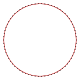{70/3}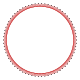{70/9}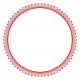{70/11}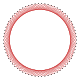{70/13}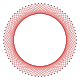{70/17}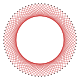{70/19} ≈164.571° ≈133.714° ≈123.429° ≈113.143° ≈92.5714° ≈82.2857°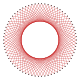{70/23}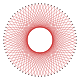{70/27}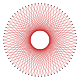{70/29}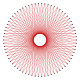{70/31}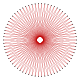{70/33} ≈61.7143° ≈41.1429° ≈30.8571° ≈20.5714° ≈10.2857°

## References

1. Greek Numbers and Numerals (Ancient and Modern) by Harry Foundalis
2. Gorini, Catherine A. (2009), The Facts on File Geometry Handbook, Infobase Publishing, p. 77, ISBN 9781438109572.
3. Constructible Polygon
4. http://www.math.iastate.edu/thesisarchive/MSM/EekhoffMSMSS07.pdf
5. The Symmetries of Things, Chapter 20